The Con-Sequences of Mind

 Author :- David A.S.Crofts Written :- December 1999 Elaboration :- April 2007

Everyone believes in the existence of “MIND”,
otherwise they could not claim to be conscious.

I propose that
“EVERYTHING” is
the expression of the thought processes of

“A MIND IN ABSOLUTE ISOLATION”
.

MIND   in   “absolute isolation”  will  REALIZE   “EVERYTHING”.

This means that the unfolding of events is “determined”,
but because the
“THE MIND IN ABSOLUTE ISOLATION”
does not know an outcome before he can deduce it,
we can be forgiven for believing that we have free will.

MIND   in   “absolute isolation”   is   logically constrained  2  follow an
ABSOLUTE
and  UNIQUE   sequence of   “discrete realizations”.

I believe that a number of religions project on to “THE MIND
IN ABSOLUTE ISOLATION”
a number of characteristics that
can best be understood as wishful thinking.

The  sequence   “goes something”   like  this  :-

I am aware that I am a  “discrete entity”

( and,  because I exist in “absolute isolation” )

(( I can only conceive of  the  “fundamental quality”  MIND ))

so

If   “there  is  2  exist”   other   “discrete entity”

( these  “discrete entity”  must  “understand”  something  I  do not )

and,  because I can conceive of  another  MIND

( understanding something I do not )

by  “understanding iteration”  I can conceive of countably infinite MIND

( understanding something I do not )

((( I   HOPE   these   MIND  “understand”  more than

they are just  “identified”  by the  NUMBER  of my iteration.)))To make it easier to write my document, from here on in,
I will pretend that I am
“THE MIND IN ABSOLUTE ISOLATION”

Please forgive my presumption.

( I  am  called  “David”)

I  can  only  “conceive”  of   “discrete entity”  that
understand  “something”  I do not.

I  can   “conceive”  of  a  countably  infinite  number of
“discrete entity”  understanding  “something”  I do not.

I  can  and  insist  upon  the  “concept”  of  a  MIND  that  can

completely understand”  all  the  “discrete entity”  I  can  “conceive” of.

( I will call her “Jenny”)

I  can  and  insist  upon  the  “conception”  of  a  countably  infinite

number of  “discrete something” where  each  “discrete something”  is

“understood”  by  only  1  “discrete entity”  and  Jenny.

By symmetry,

I   “conceive”  of   a  “discrete something”  as  being

1)       What  was  “understood”  as  MIND  “completely understands”
a  “discrete entity”.

2)       What  was  “understood”  as  MIND  “completely understands”
a  “discrete entity”.

( Mind 1      cannot  “understand”  other  “discrete entity”
because  then  a  “discrete something”  would  be
“understood” by  more  than  1  “discrete entity”
and  “Jenny”.)

( Mind 2      cannot  be  me  because  a  “discrete entity”

“understands” “something” I do not,
so it can only be  “Jenny”.)

Note :-         a  “discrete entity” is a  MIND  that  I  can  “conceive” of
that  “understands”  something  I do not.The following documents are my attempt to duplicate the thinking
of
“THE MIND IN ABSOLUTE ISOLATION” as he theorizes
himself
“understanding” another “discrete entity”.

He knows this is impossible but he will deduce that there are
dire consequences resulting from of his capacity to
“insist”.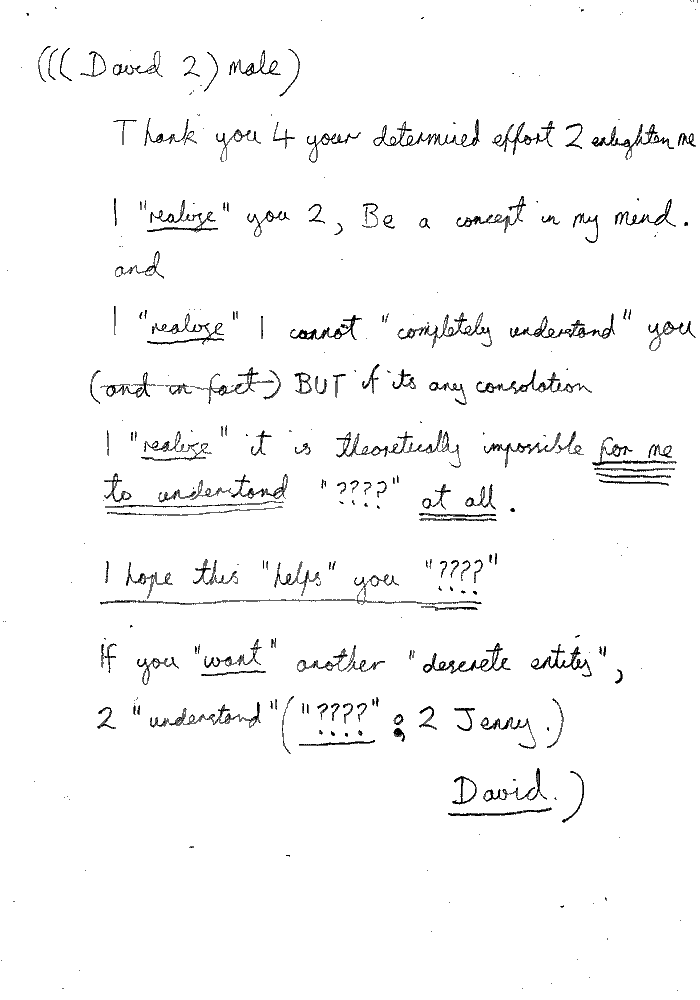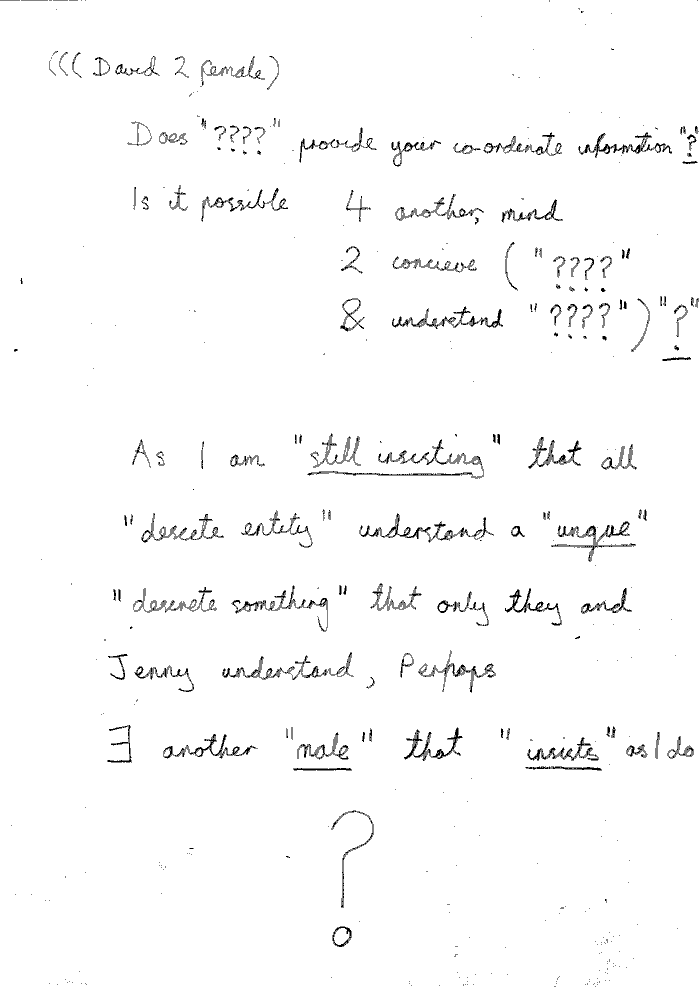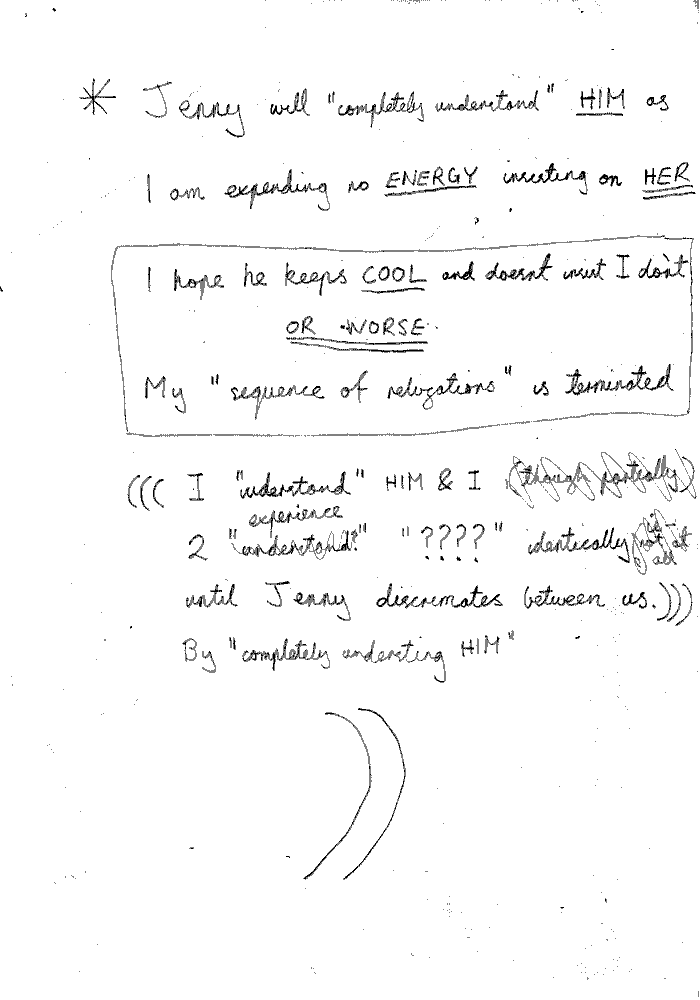By  symmetry  :-

A  “discrete something”  consists  of  :-

2   “discrete  sequence  of   discrete  realizations”

"logically constrained”  to be  “identical”.

“both”    “discrete sequence”  of  “discrete realizations”

“can be”    “understood”
“as”           “understanding”
“that”         “understands”

1)     I   am   4( “bidden”  2( “insist”  2( 2  discrete “????” ))).

“????” is a packet of information that a “MIND” can understand.

“????” can be thought of as being a discrete entity

I can conceive of the existence of two discrete
“????”.
I am forbidden to insist on the existence of two discrete
“????”.

2)    Our   “discrete sequence”  of   “discrete  realizations”
consists  “only”  of  the  “discrete realization”  :-

( 2  discrete “????” )   realize
( 4  discrete “????” )   understood   as   ( 2 discrete “????” )

The conception of two discrete “????”
leads to the conception of four discrete
“????”
understood to be possessing one of two discrete
“quality”.

Mind of  “discrete entity” )

“I understand ????”

Jenny )

“I realize that you are a “discrete entity” conceived by “David”
understanding “????” which is absolutely unique to you!”As   I   am    4(  “bidden”  2(  “insist”  2(  “????” )))   :-

I   can   only   “conceive”    2( “????” )  as  :-

2 ( 2 discrete “????” )

and  realize

2 (( 4 discrete “????” )  understood  as  ( 2 discrete “????” ))

and  conceive

( 2 discrete (( 4 discrete “????” ) understood as ( 2 discrete “????” )))

and  realize

( 4 discrete (( 4 discrete “????” ) understood as ( 2 discrete “????” ))
understood as ( 2 discrete “????” ))

as  understanding

there  can  only  “exist”

4  discrete  “fundamental” (quality)   able  to  “exist”   in
2  discrete  “fundamental” (way).

or

2  discrete  “fundamental” (way)
2 ( “exist” (4  discrete  “fundamental” (quality)).

or

(2  discrete  “fundamental” (way))   “exist”
(4  discrete  “fundamental” (quality)).4  discrete  “fundamental” (quality)    are  able  to  “exist”   in
2  discrete  “fundamental” (way).

“fundamental” (way) :-

1)      ( “????” )                      :-       by  “????”  conceiving

2)      OUT ( “????” )             :-       by  “????”  understanding

“fundamental” (quality) :-

1)      OUT

:-       “????”   can  conceive                                    “????”

2)      OUT ( “????” )

:-       “????”   can  understand                           2 ( “????” )

3)      OUT (  2  ( “????” ))

:-       “????”   can   understand         ( 2  discrete  “????” )

4)      OUT (  4  ( “????”  ))

:-       “????”   can   conceive         2  ( 2  discrete  “????” )The following is a more detailed description of the

“fundamental” (quality).

“????”  is the packet of information or “discrete entity”
that possesses the specified
“fundamental” (quality).

“????”  is an arbitrary packet of information or “discrete entity”.

“fundamental” (quality) :-

1)      OUT

:-       “????”   can  conceive                                 “????”

2)      OUT ( “????” )

:-       “????”   can  understand
the  combined  information
of  two  instances  of
“????”

3)      OUT (  2  ( “????” ))

:-       “????”   can  understand
the  existence  of  two  discrete
“????”

4)      OUT (  4  ( “????”  ))

:-       “????”   can  conceive
the  combined  information
of  two  discrete  instances  of
“????”I    “believe”   MIND   can   “possess”    the   4  discrete  “fundamental” (quality)

by   either   conception  or  understanding.

(  The Fundamental Quality  3  )   is  :-

OUT( 2 ( “????”) )      can understand        ( 2  discrete “????” )

“I  can”  conceive  “I  can  only  understand”       ( 2  discrete  “????” )   and

proudly  proclaim  them  to  be   :- “ (

1)      OUT( 2 ( MALE ) )

2)      OUT( 2 ( FEMALE ) )

and  understand  these  ( 2  discrete  “????” )  as  understanding    :- (

1)      4 ( “bidden”  2 ( “????” ) )      By     “????”      understanding

2)      4 ( “bidden”  2 ( “????” ) )      By     “????”      conceiving

) -:

)” -:

Note :-         ( 2  discrete “????” )   realize
( 4  discrete “????” )   understood   as   ( 2 discrete “????” )These :-       ( 2  discrete “????” )   realize
( 4  discrete “????” )   understood   as   (  MALE  &  FEMALE  )

The   (  “four  discrete  fundamental  long”  )   :- “(

1)      “????”        “realizing”  -

:-       ( 2  “permit”  2 )           or

:-       OUT( 2 ( FEMALE ) )            or

:-       4 (  “bidden”  2 (  “insist”  2 (  “????” )))

2)      “????”        “realizing”  -

:-       ( 4  “bidden”  2 )           or

:-       OUT( 2 ( MALE ) )                 or

:-       4 (  “bidden”  2 (  “insist”  2 (  “????” )))

3)      OUT( 2 ( MALE ) )                 “realizing”  -

:-       ( 4  “bidden”  2  only )            or

:-       OUT           or

:-       4 (  “bidden”  2 (  “insist”  2 ( 2  discrete  “????” )))

4)      OUT( 2 ( FEMALE ) )            “realizing”  -

:-       ( 2  “permit”  only  2 )             or

:-       OUT           or

:-       4 (  “bidden”  2 (  “insist”  2 ( 2  discrete  “????” )))

)” -:I    “understand”   the   (  “four  discrete  fundamental  long”  )

as   “understanding”  :-

( A   discrete  “????” )    is  a   “consequence”   of    ( A  discrete   “????” )

being   “completely understood”   by :-

OUT( 2 ( FEMALE ) )  &  OUT( 2 ( MALE ) )

( 2   discrete  “????” )    is  a   “consequence”   of    ( A  discrete   “????” )

being   “completely understood”   by :-

OUT( 2 ( FEMALE ) )  &  OUT( 2 ( MALE ) )  &  Jenny

( A  discrete   “????” )

“is”                “logically connected”   with   ( 2  discrete  “????” )
by  these   ( 2  discrete  “????” )

“being”          “understood”
“as”               “understanding”
“that”             “understands”

( 2   “permit”  only   2 )

4 (  “bidden”  2 (  “insist”  2 (  2  discrete  “????” )))

( 4   “bidden”  2  only

by   :-   Jenny.( A  discrete   “????” )  is   “logically connected”   with   ( 2  discrete  “????” )

( A  discrete   “????” )  is  :-

“information”   that   represents  the

“life  experience”  of   these   ( 2  discrete  “????” )   as   a

“consolidated  memory”

made  by   Jenny   “completely  understanding”   them.

A   “life  experience”    as    A   “consolidated  memory”    is  :-

A   finite  set  containing  :-

A   countable  number  of   “connection”   between   2   “discrete entity”.

Note :-     There  exists  only   2   class  of   “connection”.

1)      “discrete entity”   conceives of   “discrete entity”  as  “????”

2)      “discrete entity”   understands    “discrete entity”  as  “????”

:-     integers  are  clearly  “discrete entity”

although   1&2 …  are  clearly  not  ( 2 discrete  “????” )

“????”   introduces   the  concepts   ( “unit” ),   (square),    (cube),  …

The       “consolidated  memory”   is  formed  by  :-

Jenny    “justly  reducing”     the  countable  number  of  “connection”

to  the  form  :-

( 2   “permit”  only   2 )

4 (  “bidden”  2 (  “insist”  2 (  2  discrete  “????” )))

( 4  “bidden”  2  only )

To make the “consolidated  memory”
the meaning of the terms
“permit”, “bidden” and “insist”
are the only packets information needed from
“ENGLISH”.

All the other terms are absolute in
“MATHEMATICS”.( MIND  is   “permitted”  2  only )  (

“insist”  in  ( the  “four  discrete  fundamental  long” ) ).

( MIND  is   “forbidden”  only  2 )  (

“insist”  in  ( the  “four  discrete  fundamental  long” ) ).

Note :-        I have reversed the only compared with the correct gender usage.
This indicates that my inquiry has now become anal.
Unfortunately, Physics is now only the study of  excrement.
Hence, I am prepared to continue.

The   UNIT  of   ( the  “four  discrete  fundamental  long” )   :- “(

1)      “????”                                   “realizing”           OUT( 2 ( FEMALE ) )

2)      “????”                                   “realizing”           OUT( 2 ( MALE ) )

3)      OUT( 2 ( MALE ) )                “realizing”           OUT

4)      OUT( 2 ( FEMALE ) )           “realizing”           OUT

1)  &  2)               (  Time      per      Distance  )

3)  &  4)               (  “????”    per     (square)    Distance  )

)”-:

The “UNITs” above  and  the “ENGLISH” terms “permit”, “bidden”
and
“insist” strongly lead me to believe I am half way to deducing

“ELECTRO-MAGNETISM”
.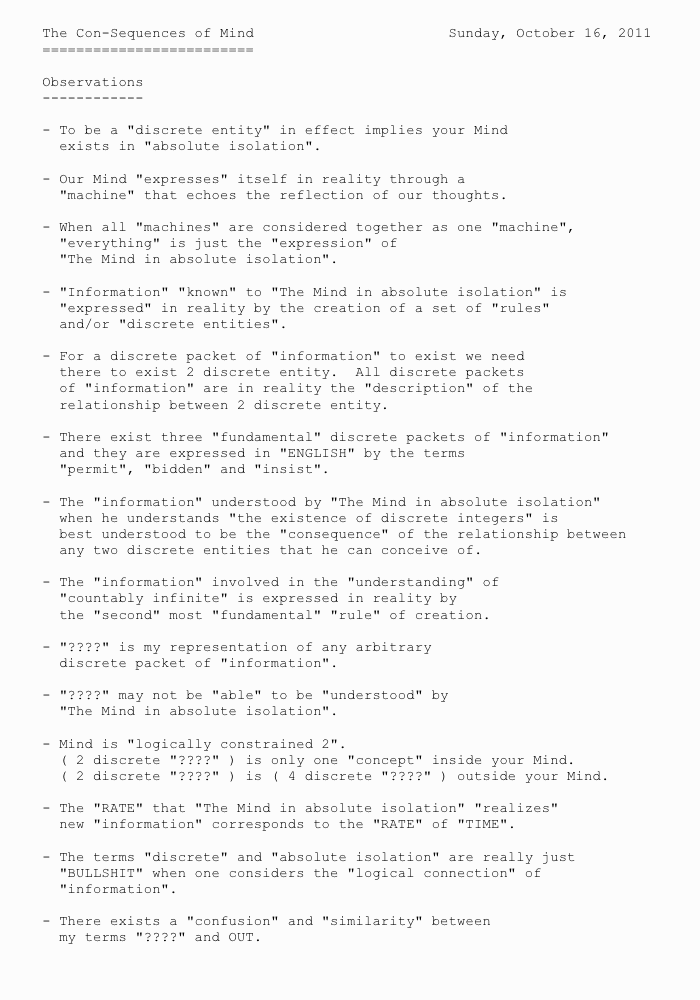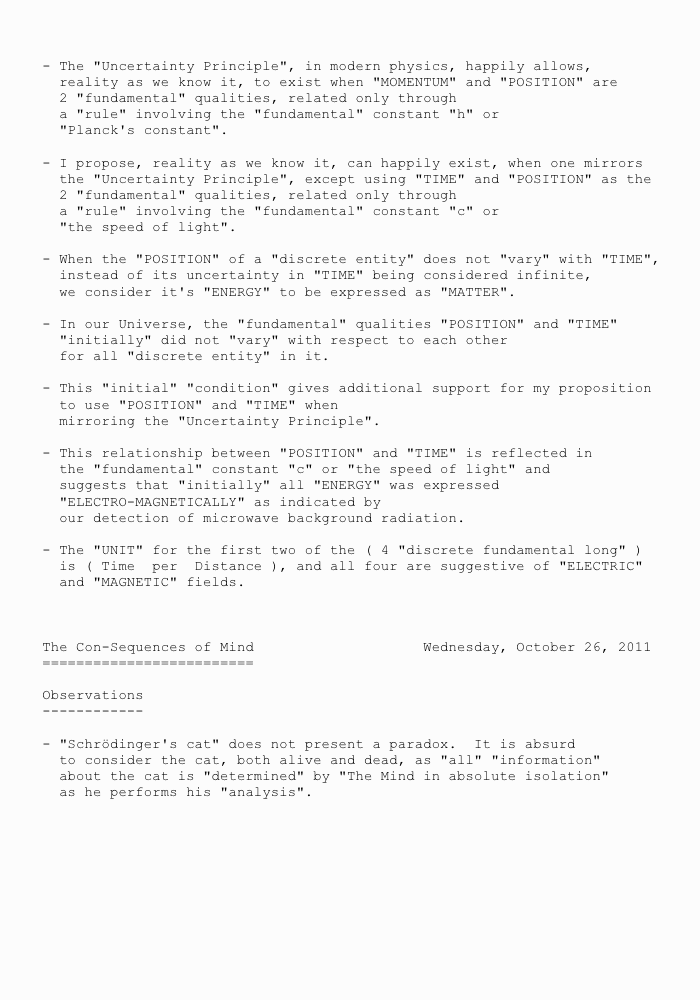THE  CONSEQUENCE  OF  2I  can  only  :-}

“conceive”  of   “discrete entity” that  understand  “something”  I do not.

{-:“I”

I understand(I understand (   ))“I can”

I understand(I understand(I can))

(I can) understand((I can) understand)

“I can

I understand(I  understand(“I can” as understanding))

((“I can”)  is not)  (4  bidden)  (2 exist)

(“I can”)  is  ((4 bidden  2)  (4 bid))  (4  bidden)  (2 exist)

(“I can”)  is  ((4  bidden)  (2 exist))  (4 bid)  (4  bidden)  (2 exist))

(“I can”)  is  ((4  bidden)  (2 exist))

(“I can”)  is   (4  bidden  2)“I can only”

“I can” understand(

“I can” understand(

(“I can” only) understand only as understanding))

(only “I can” )   (“I can” only)

(only (4  bidden  2))   ((4  bidden  2) only)

“4 bidden  2 only (????)”

“????”

“I  can  only”  understand (2(  ))“I can only conceive”

“????   4  bidden  2  (only  “????”)”

“4  bidden  2  only  (“????”)”    “4  bidden  only   2  (“????”)”

““????””    ““????””

4  bidden  2  only  “????”

4  bidden  2  only  understand   2(  )  2(  )

4  bidden  2  only  understand   2(  )  2(  )

4  bidden  2  understand  only  ( I can )

“I can”  conceive  “I can only understand”  :-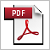a_matter_of_crap.pdfbi_tarian.pdfcon_mind.pdfher_hard_on_circling_not_box.pdfquantum_computing.pdfthe-boundary-of-helen.pdfunion_minds.pdf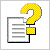David Crofts' Page